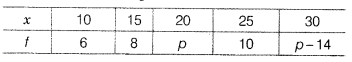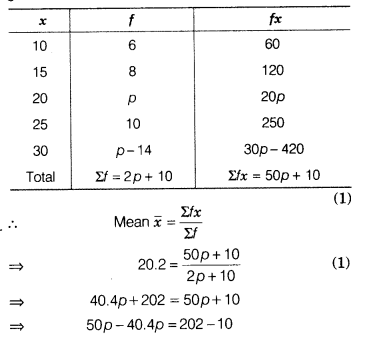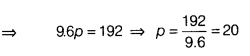# If the mean of the given data is 20.2, then find the value of p

If the mean of the given data is 20.2, then find the value of p.The product of observations and its frequencies are given belowFirstly, draw two perpendicular llines.